# Generalized derivative

of function type

An extension of the idea of a derivative to some classes of non-differentiable functions. The first definition is due to S.L. Sobolev (see , ), who arrived at a definition of a generalized derivative from the point of view of his concept of a generalized function.

Let $f$ and $\phi$ be locally integrable functions on an open set $\Omega\subset \mathbb R^n$, that is, Lebesgue integrable on any closed bounded set $F\subset\Omega$. Then $\phi$ is the generalized derivative of $f$ with respect to $x_j$ on $\Omega$, and one writes $\phi = \frac{\partial f}{\partial x_j}$ (or $\phi = D_jf$), if for any infinitely-differentiable function $\psi$ with compact support in $\Omega$ (see Function of compact support)

\begin{equation}\label{eq:2} \int\limits_{\Omega}f(x)\frac{\partial \psi}{\partial x_j}(x)\,dx = -\int\limits_{\Omega}\phi(x) \psi(x)\,dx. \end{equation}

Generalized derivatives of a higher order $D^{\alpha}_xf$ are defined as follows.

\begin{equation}\label{eq:1} \int\limits_{\Omega}f(x)D^{\alpha}_x\psi(x)\,dx = (-1)^{|\alpha|}\int\limits_{\Omega}\phi(x) \psi(x)\,dx, \end{equation}

where multiindex $\alpha = (\alpha_1,\dots,\alpha_n)$, $x=(x_1,\dots,x_n)$, $|\alpha| = \alpha_1+\dots+\alpha_n$ and differential operator $D^{\alpha}_x$ is just short notation for $\frac{\partial^{\alpha_1+\dots+\alpha_n}}{\partial x_1^{\alpha_1}\dots\partial x_n^{\alpha_n}}$. In this case $\phi = D^{\alpha}_xf$ is $n$-th generalized derivatives of function $f$.

Another equivalent definition of the generalized derivative $\frac{\partial f}{\partial x_j}$ is the following. If $f$ can be modified on a set of $n$-dimensional measure zero so that the modified function (which will again be denoted by $f$) is locally absolutely continuous with respect to $x_j$ for almost-all (in the sense of the $(n-1)$-dimensional Lebesgue measure) $x^j=(x_1,\dots,x_{j-1},x_{j+1},\dots,x_n)$ belonging to the projection $\Omega^j$ of $\Omega$ onto the plane $x_j=0$, then $f$ has partial derivative (in the usual sense of the word) $\frac{\partial f}{\partial x_j}$ almost-everywhere on $\Omega$. If a function $\phi = \frac{\partial f}{\partial x_j}$ almost-everywhere on $\Omega$, then $\phi$ is a generalized derivative of $f$ with respect to $x_j$ on $\Omega$. Thus, a generalized derivative is defined almost-everywhere on $\Omega$ if $f$ is continuous and the ordinary derivative $\frac{\partial f}{\partial x_j}$ is continuous on $\Omega$, then it is also a generalized derivative of $f$ with respect to $x_j$ on $\Omega$.

There is the third equivalent definition of a generalized derivative. Suppose that for each closed bounded set $F\subset\Omega$, the functionsand, defined on, have the properties: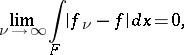and suppose that the functions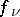,and their partial derivatives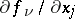are continuous on. Thenis the generalized partial derivative ofwith respect to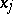on(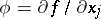) (see also Sobolev space).

From the point of view of the theory of generalized functions, a generalized derivative can be defined as follows: Suppose one is given a functionthat is locally summable on, considered as a generalized function, and let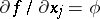be the partial derivative in the sense of the theory of generalized functions. Ifrepresents a function that is locally summable on, thenis a generalized derivative (in the first (original) sense).

The concept of a generalized derivative had been considered even earlier (see  for example, where generalized derivatives with integrable square onare considered). Subsequently, many investigators arrived at this concept independently of their predecessors (on this question see ).

How to Cite This Entry:
Generalized derivative. Encyclopedia of Mathematics. URL: http://encyclopediaofmath.org/index.php?title=Generalized_derivative&oldid=28804
This article was adapted from an original article by S.M. Nikol'skii (originator), which appeared in Encyclopedia of Mathematics - ISBN 1402006098. See original article